Ask your WordPress questions! Pay money and get answers fast!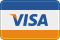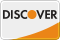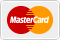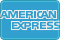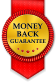# post__in() array as argument isn't formatted right WordPress

• SOLVED

I'm building a WP_Query with the following \$args:

\$args = array(
'post__in' => array (5, 6, 7),
'post_type' => 'listing',
'post_status' => 'publish'
);

\$loop = new WP_Query( \$args );

****************

Here the 'post__in' has hard coded values in the array. This works fine. But in reality, this list of numbers comes from \$_POST

For example, the \$_POST['ids'] value is "5, 6, 7".

If my arg is:

'post__in' => array(\$_POST['ids'])

The array becomes associative, for example:

array(=>5 =>6 =>7)

For some reason my app doesn't like this kind of array. It will ONLY work if the array is in the format like: array (5, 6, 7)

I can't figure out how to force the array to be in this simple kind of format.

****************

NOTE: in normal circumstances I can pass an array just fine in the format of array(=>5 =>6 =>7), but in this particular case the \$args are getting made in AJAX code. (infinite scrolling of WP post results). Again, if the array is hard coded with comma delimited numbers it works fine.

2016-09-13

Try this:

``` 'post__in' => array_map( 'absint', array_values( \$_POST['ids'] ) ) ```

Note that you should in any case sanitize input value before querying database with it.

Thanks for your fast response. But it didn't work....

... that said strangely when I print_r this:

print_r (array(\$_POST['ids']));

I now get an array like this:

Array (  => 5, 6, 7 )

I'm not sure how before I was getting the different style of array. Anyway, assuming this is what get's output, how to get it in a format like this?

array(5, 6, 7)

Thanks!

Could you please var_dump value of \$_POST['ids'] without any change, like var_dump( \$_POST['ids'] )? It looks like this is a string and explode should be used, we just need to know delimiters, something like:

``` 'post__in' => array_map( 'absint', array_values( explode( ', ', \$_POST['ids'] ) ) ) ```

That code works, thanks so much!!!

Note that if I do this:

print_r (array_map( 'absint', array_values( explode( ', ', \$_POST['ids'] ) ) ));

it outputs the array in the "bad" way. But somehow I'm getting the correct output in the WP loop. Strange. Thanks so much!!